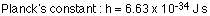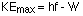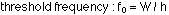## Electrons, photons, and the photo-electric effect

8-4-00

Sections 27.1 - 27.3

We're now starting to talk about quantum mechanics, the physics of the very small.

### Planck's constantAt the end of the 19th century one of the most intriguing puzzles in physics involved the spectrum of radiation emitted by a hot object. Specifically, the emitter was assumed to be a blackbody, a perfect radiator. The hotter a blackbody is, the more the peak in the spectrum of emitted radiation shifts to shorter wavelength. Nobody could explain why there was a peak in the distribution at all, however; the theory at the time predicted that for a blackbody, the intensity of radiation just kept increasing as the wavelength decreased. This was known as the ultraviolet catastrophe, because the theory predicted that an infinite amount of energy was emitted by a radiating object.

Clearly, this prediction was in conflict with the idea of conservation of energy, not to mention being in serious disagreement with experimental observation. No one could account for the discrepancy, however, until Max Planck came up with the idea that a blackbody was made up of a whole bunch of oscillating atoms, and that the energy of each oscillating atom was quantized. That last point is the key : the energy of the atoms could only take on discrete values, and these values depended on the frequency of the oscillation:

Planck's prediction of the energy of an oscillating atom : E = nhf (n = 0, 1, 2, 3 ...)
where f is the frequency, n is an integer, and h is a constant known as Planck's constant. This constant shows up in many different areas of quantum mechanics.The spectra predicted for a radiating blackbody made up of these oscillating atoms agrees very well with experimentally-determined spectra.

Planck's idea of discrete energy levels led Einstein to the idea that electromagnetic waves have a particle nature. When Planck's oscillating atoms lose energy, they can do so only by making a jump down to a lower energy level. The energy lost by the atoms is given off as an electromagnetic wave. Because the energy levels of the oscillating atoms are separated by hf, the energy carried off by the electromagnetic wave must be hf.

### The photoelectric effect

Einstein won the Nobel Prize for Physics not for his work on relativity, but for explaining the photoelectric effect. He proposed that light is made up of packets of energy called photons. Photons have no mass, but they have momentum and they have an energy given by:

Energy of a photon : E = hf

The photoelectric effect works like this. If you shine light of high enough energy on to a metal, electrons will be emitted from the metal. Light below a certain threshold frequency, no matter how intense, will not cause any electrons to be emitted. Light above the threshold frequency, even if it's not very intense, will always cause electrons to be emitted.

The explanation for the photoelectric effect goes like this: it takes a certain energy to eject an electron from a metal surface. This energy is known as the work function (W), which depends on the metal. Electrons can gain energy by interacting with photons. If a photon has an energy at least as big as the work function, the photon energy can be transferred to the electron and the electron will have enough energy to escape from the metal. A photon with an energy less than the work function will never be able to eject electrons.

Before Einstein's explanation, the photoelectric effect was a real mystery. Scientists couldn't really understand why low-frequency high-intensity light would not cause electrons to be emitted, while higher-frequency low-intensity light would. Knowing that light is made up of photons, it's easy to explain now. It's not the total amount of energy (i.e., the intensity) that's important, but the energy per photon.

When light of frequency f is incident on a metal surface that has a work function W, the maximum kinetic energy of the emitted electrons is given by:Note that this is the maximum possible kinetic energy because W is the minimum energy necessary to liberate an electron. The threshold frequency, the minimum frequency the photons can have to produce the emission of electrons, is when the photon energy is just equal to the work function: